##### Physics I: 501 Practice Problems For Dummies (+ Free Online Practice)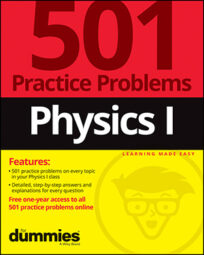In physics, you can relate the work done on an object and the change in the object's kinetic energy. For example, if two people push identical shopping carts, but one person does more work, you can calculate how much faster their cart will go than the other.

Here are some practice questions that you can try.

## Practice questions

1. Sam and Eric race each other while each is pushing a 12-kilogram shopping cart from rest, but Sam performs three times the amount of work as Eric. If Eric's speed after 5 seconds is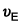what is Sam's speed after 5 seconds? Ignore friction and give your answer as an exact multiple of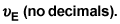2. An engine provides 500 joules of work to change a car's kinetic energy from 220 joules to 670 joules. How much energy is lost to friction?

3. Sammy catches a 480-gram baseball traveling 130 kilometers per hour, bringing it to a complete stop in 0.8 seconds. How many joules of work does he do on the ball?

The following are the answers to the practice questions:

1.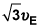The net work performed on an object is equal to the object's change in kinetic energy. Given that each racer's initial velocity is 0 meters per second, this reduces to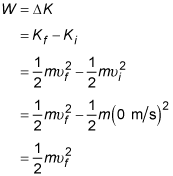In this case, work is proportional to the square of the final velocity. If work is tripled, so too is the square of the final velocity. Or, removing the square, if work is multiplied by three, the velocity is multiplied by the square root of three.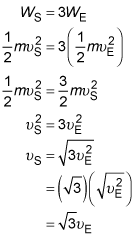2. 50 J

The work-energy theorem states that the net amount of work done on an object is equal to the object's final kinetic energy minus its initial kinetic energy. Both the engine and friction do work on the car; the sum of that work must be equal to the change in the car's kinetic energy.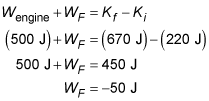Friction does 50 joules of work; the negative sign indicates that the force of friction points in the opposite direction from the car's motion — which is always the case with frictional forces.

3. –312.8 J

First convert all the units given into "correct" SI (International System of Units) form: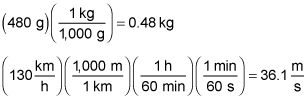The work-energy formula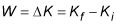where K represents kinetic energy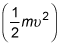states that work done on an object changes its kinetic energy. Use the formula to solve for the work done on the baseball: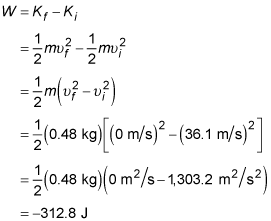The negative sign indicates that the force used to stop the ball and the distance the ball moves while that force is exerted are in opposite directions, which is indeed the case.